# IEnumerable and IQueryable in C#

## IEnumerable and IQueryable in C# with Examples

In this article, I am going to discuss IEnumerable and IQueryable in C# with Examples. Please read our previous article where we discussed the different ways to write LINQ queries with Examples. In this article, we are going to discuss the following concepts in detail.

1. What is IEnumerable in C#?
2. What is IQueryable in C#?
3. Examples of IEnumerable and IQueryable

In our previous article, we write the following program using LINQ Query Syntax.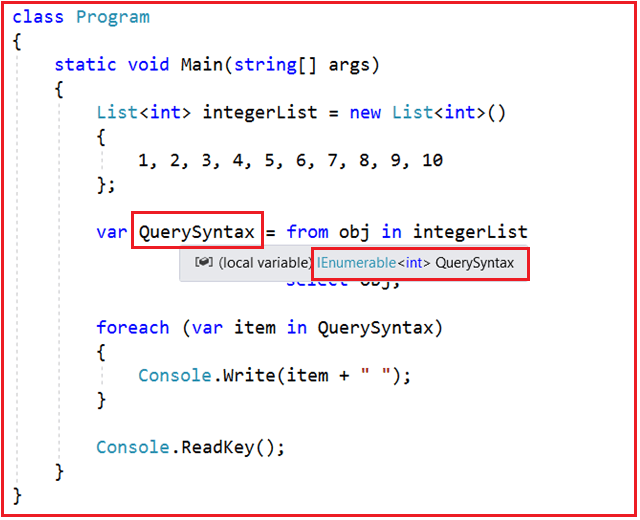In the above example, we use the var keyword to create the variable and store the result of the LINQ query. So let’s check what is the type of the variable. In order to check this, just mouse over the pointer onto the QuerySynntax variable and you will see that the type is IEnumerable<int> which is a generic type. So it is important to understand what is IEnumerable<T>.

So in the above example, instead of writing the var keyword, you can also write IEnumerable<int> and it should work as expected as shown in the below example.

```using System;
using System.Collections.Generic;
using System.Linq;

namespace LINQDemo
{
class Program
{
static void Main(string[] args)
{
List<int> integerList = new List<int>()
{
1, 2, 3, 4, 5, 6, 7, 8, 9, 10
};

IEnumerable<int> QuerySyntax = from obj in integerList
where obj > 5
select obj;

foreach (var item in QuerySyntax)
{
Console.Write(item + " ");
}

}
}
}```

With this kept in mind, let us understand what is IEnumerable.

##### What is IEnumerable in C#?

Let us first see the definition of the IEnumerable which is given below.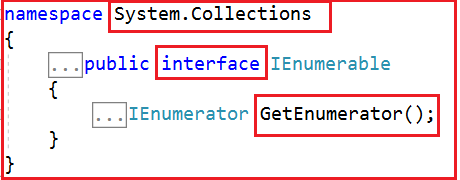As we see from the above image, IEnumerable is an interface that is available in System.Collection namespace. The IEnumerable interface is a type of iteration design pattern. It means we can iterate on the collection of the type IEnumerable. As you can see in the above definition, the IEnumerable interface has one method called GetEnumerator which will return an IEnumerator that iterates through a collection.

The IEnumerable interface has also a child generic interface i.e. IEnumerable<T>. Let’s see the definition of the IEnumerable<T> interface.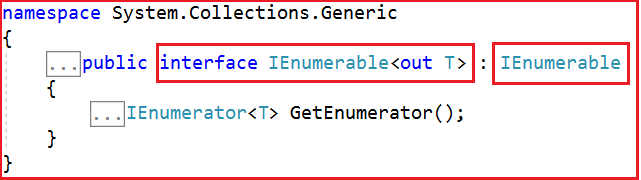The most important point that you need to remember is, in C#, all the collection classes (both generic and non-generic) implement the IEnumerable interface. Let us prove this by visiting the definition of List<T> generic collection class as shown in the below image.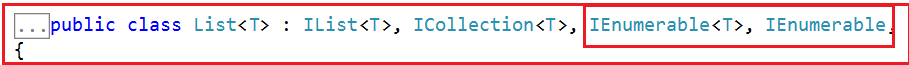Now let us see the definition of the ArrayList collection which is a non-generic collection class.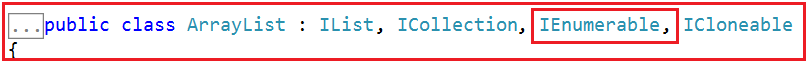##### Example to understand IEnumerable<T> with Complex Type using C#.

Whenever we want to work with in-memory objects, then we need to use the IEnumerabe interface and the reason for this will be discussed in our next article. In the below example, we have a complex type called Student. Within the Main method, we have created a collection to hold a list of students. Then our requirement is to display only the Male students. And for this, we are using the LINQ Query to fetch the Male students from the student collection data source, and then using a for each loop we are iterating through the IEnumerable<Student> collection.

```using System;
using System.Collections.Generic;
using System.Linq;

namespace LINQDemo
{
class Program
{
static void Main(string[] args)
{
List<Student> studentList = new List<Student>()
{
new Student(){ID = 1, Name = "James", Gender = "Male"},
new Student(){ID = 2, Name = "Sara", Gender = "Female"},
new Student(){ID = 3, Name = "Steve", Gender = "Male"},
new Student(){ID = 4, Name = "Pam", Gender = "Female"}
};

//Linq Query to Fetch all students with Gender Male
IEnumerable<Student> QuerySyntax = from std in studentList
where std.Gender == "Male"
select std;
//Iterate through the collection
foreach (var student in QuerySyntax)
{
Console.WriteLine( \$"ID : {student.ID}  Name : {student.Name}");
}

}
}

public class Student
{
public int ID { get; set; }
public string Name { get; set; }
public string Gender { get; set; }
}
}```

When we execute the program it will display the result as expected as shown in the below image.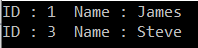##### What is IQueryable in C#?

Let us first see the definition of IQueryable as shown below.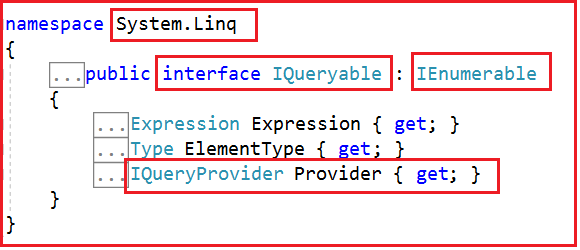As you can see in the above image, the IQueryable is an interface and it is available in System.Linq namespace. The IQuerable interface is a child of the IEnumerable interface. So we can store IQuerable in a variable of type IEnumerable. The IQuerable interface has a property called Provider which is of type IQueryProvider interface. Let us see the definition of IQueryProvider.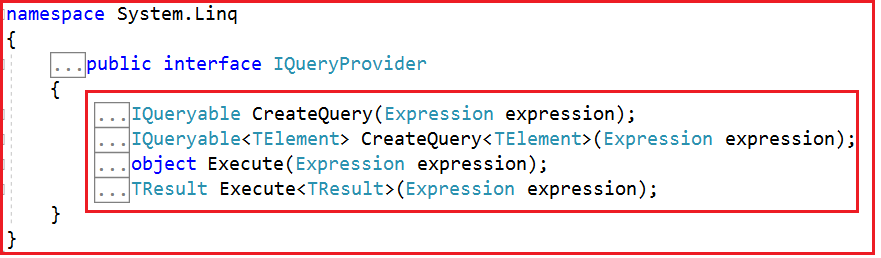The methods provided by the IQueryProvider are used to create all Linq Providers. So, this is the best choice for other data providers such as Linq to SQL, Linq to Entities, etc. Why we will discuss this in our next article.

##### Example to understand IQueryable<T> Interface in C#.

The following is the same example as the previous one. Here, instead of IEnumerable<Student>, we are storing the result in IQuerable<Student> variable. To store the result in the IQuerable<Student> variable, we need to call the AsQueryable() method on the data source.

```using System;
using System.Collections.Generic;
using System.Linq;

namespace LINQDemo
{
class Program
{
static void Main(string[] args)
{
List<Student> studentList = new List<Student>()
{
new Student(){ID = 1, Name = "James", Gender = "Male"},
new Student(){ID = 2, Name = "Sara", Gender = "Female"},
new Student(){ID = 3, Name = "Steve", Gender = "Male"},
new Student(){ID = 4, Name = "Pam", Gender = "Female"}
};

//Linq Query to Fetch all students with Gender Male
IQueryable<Student> MethodSyntax = studentList.AsQueryable()
.Where(std => std.Gender == "Male");

//Iterate through the collection
foreach (var student in MethodSyntax)
{
Console.WriteLine( \$"ID : {student.ID}  Name : {student.Name}");
}

}
}

public class Student
{
public int ID { get; set; }
public string Name { get; set; }
public string Gender { get; set; }
}
}```

Now run the application and you will see the data as expected as shown in the below image.The point that you need to remember is in order to return a collection of type IQueryable, first, you need to call the AsQueryable() method on the data source as we did in the above example.

In the next article, I am going to discuss the Differences Between IEnumerable and IQueryable in C# and when to use one over another with an example. I hope this article gives you a very good understanding of two important interfaces i.e. IEnumerable and IQueryable in C# with Examples.

### 2 thoughts on “IEnumerable and IQueryable in C#”

1.2.Since my 3 year of Experience , Now me all doubt is clear regarding this IEnumerable and IQueryable.
Thanks ! a lot for this.